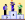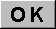Advanced Chemistry QuizzQuizz "Physics, Chemistry" - 10 questionstotal = 377 player(s)

Question 1 / 10
 What can you find in car battery ? Lead and sulfuric acid Gold and acetic acid Uranium and Silver No idea None correct answerQuestion 2 / 10
 Which element makes green color of fireworks Cesium Barium Copper No idea None correct answerQuestion 3 / 10
 What is the formula of the perfect gas ? PV=nRT AB�=AC�+BC� E=mc� No idea None correct answerQuestion 4 / 10
 What is the name of the third isotope of Hydrogen Helium Deuterium Tritium No idea None correct answerQuestion 5 / 10
 What is the formula of stainless steel ? Carbon, Iron, Copper Carbon, Iron, Chromium Carbon, Iron No idea None correct answerQuestion 6 / 10
 Tick the right answer HCl + H2O -> H+ + Cl- HCl+ H2O -> H3OCl HCl + H2O -> H3O+ + Cl- No idea None correct answerQuestion 7 / 10
 Tick the right answer CH3NH2 + CH3COOH -> CH3NH3+ + CH3OO- CH3NH2 + CH3COONa -> CH3Na + CH3COONH2 CH3NH2 + CH3COOH -> H2O + NH3 + x kJ No idea None correct answerQuestion 8 / 10
 Gold can be dissolved in sulfuric acid Yes Yes but with water added Only in chlorhydric acid No idea None correct answerQuestion 9 / 10
 What is the Aqua regia formula HCl + H2SO4 HNO3 + 3 HCl NH4OH + HCl No idea None correct answerQuestion 10 / 10
 Tick the right name of NaHCO3 Carbonic acid of sodium Calcium hydrogencarbonate Sodium hydrogencarbonate No idea None correct answer.
End of the game
Now, let's calculate and register your score.